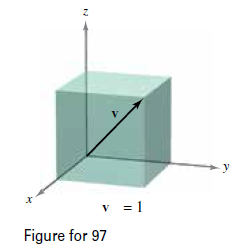Chapter 11.2, Problem 99E

Chapter
Section
Textbook Problem

# Diagonal of a Cube Find the component form of the unit vector v in the direction of the diagonal of the cube shown in the figure.To determine

To calculate: The component form of unit vector v in the direction of diagonal of cubeshown in figure.

Explanation

Given:

v=1 andunit vector v in the direction of diagonal of cube is shown in figure

Please Refer the Graphs in the given question.

Formula used:

Magnitude of vector formula

Calculation:

We have to find the component form of unit vector v in the direction of diagonal of cube.

We know that length of diagonal of cube of side ‘a’ is given by a3.

Here length of diagonal is given as v=1.

Then after equating the both, we obtain

1=a3a=13

Consider the figure below,

Then coordinates of point A (of diagonal OA) are: (13,13,13)

Then the vector has initial component (

### Still sussing out bartleby?

Check out a sample textbook solution.

See a sample solution

#### The Solution to Your Study Problems

Bartleby provides explanations to thousands of textbook problems written by our experts, many with advanced degrees!

Get Started